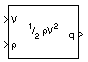# Dynamic Pressure

Compute dynamic pressure using velocity and air density

• Library:
• Aerospace Blockset / Flight Parameters

•## Description

The Dynamic Pressure block computes dynamic pressure.

Dynamic pressure is defined as:

`$\overline{q}=\frac{1}{2}\rho {V}^{2},$`

whereis air density and V is velocity.

## Ports

### Input

expand all

Velocity, specified as a three-element vector.

Data Types: `double`

Air density, specified as a scalar.

Data Types: `double`

### Output

expand all

Dynamic pressure, returned as a scalar.

Data Types: `double`

## Version History

Introduced before R2006a